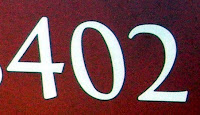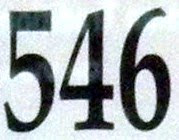## Friday, May 28, 2010

### 50

50 = 2 x 52.

50 = 8 + 9 + 10 + 11 + 12.

50 can be expressed as a sum of primes in two distinct ways such that all primes involved are consecutive: 50 = 2 + 5 + 7 + 17 + 19 = 3 + 11 + 13 + 23.

50 is the smallest number with two representations as a sum of two squares: 50 = 12 + 72 = 52 + 52.

50 is 1212 in base 3, 101 in base 7, and 55 in base 9.The flag of the United States of America has 50 stars representing its 50 constituent states. If you count stars along the diagonals, you get the sum 1 + 3 + 5 + 7 + 9 + 9 + 7 + 5 + 3 + 1 = 50.

## Thursday, May 27, 2010

### 352

352 = 25 x 11.

352 is the only number consisting of three distinct prime digits such that the first and third equal the third raised to the power of the second: 32 = 25.

352 has a unique representation as a sum of three squares: 352 = 82 + 122 + 122.

352 is the maximum number of volumes into which 11 intersecting spheres can divide space.

352 is the maximum number of regions into which 26 lines can divide a plane.352 is the number of n-Queens Problem solutions for n = 9.

## Wednesday, May 26, 2010

### 226

226 = 2 x 113.

226 has a representation as a sum of two squares: 226 = 12 + 152.

2242 and 2262 are the squares of two consecutive even numbers, where the squares each use the same digits: 2242 = 50176 and 2262 = 51076.

226 is 1401 in base 5 and 1014 in base 6.Radium-is a radioactive decay product of uranium-238; radium-226 itself decays to form the inert gas radon-222.

Source: Numeropedia

## Tuesday, May 25, 2010

### 950

950 = 2 x 52 x 19.

950 is the generalized Catalan number C(17, 3).

950 is 12300 in base 5, 4222 in base 6, 2525 in base 7, and 1666 in base 8.950 KJR is Seattle's all sports radio station.

## Monday, May 24, 2010

### 510

510 = 2 x 3 x 5 x 17.

The concatenation of 510 with itself (510510) is the product of the first seven primes (2 x 3 x 5 x 7 x 11 x 13 x 17) and also the product of the seventh through tenth Fibonacci numbers (13 x 21 x 34 x 55).

510 is the number of binary rooted trees with 14 vertices.

510 is 626 in base 9, 424 in base 11, and 303 in base 13.510 Mabella is an asteroid discovered in 1903.

## Friday, May 21, 2010

### 4329

4329 = 32 x 13 x 37.

4329 has two representations as a sum of two squares: 4329 = 272 + 602 = 452 + 482.

4329 is a divisor of 1003 - 1.

4329 is the only number n such that n, 2n, 4n, and 6n together contain every digit from 1 to 9 exactly twice: 4329, 8658, 17316, 25974.IC4329 is a supercluster of galaxies found in the constellation Centaurus.

## Thursday, May 20, 2010

### 402

402 = 2 x 3 x 67. As the product of three distinct primes, 402 is a sphenic number.

402 is the number of graphs with eight vertices and nine edges.

402 is 123 in base 19.402 is the telephone area code for Omaha, Nebraska.

## Wednesday, May 19, 2010

### 953

953 is a prime number.

953 is a Sophie Germain prime because 2 x 953 + 1 = 1907 is also a prime.

953 has a representation as a sum of two squares: 953 = 132 + 282.

953 is the hypotenuse of a primitive Pythagorean triple: 9532 = 6152 + 7282.

953 is the largest prime factor of 54321.953 Painleva is a main-belt asteroid discovered in 1921.

## Tuesday, May 18, 2010

### 432

432 = 24 x 33.

432 = 4 x 33 x 22.

432 is the sum of four consecutive primes: 432 = 103 + 107 + 109 + 113.

432 +/- 1 are twin primes. Note the descending digits.

432 is 12300 in base 4, 2000 in base 6, 660 in base 8, 363 in base 11, and 300 in base 12.432 is sometimes known as the "cycle of time" number.

## Monday, May 17, 2010

### 760

760 = 23 x 5 x 19.

760 has the unique representation 760 = 62 + 182 + 202 as a sum of three squares.

760 is the number of integer partitions of 37 into distinct parts.

760 is a centered triangular number: 3n(n - 1)/2 + 1, for n = 23.Telephone area code 760 covers eastern southern California.

## Friday, May 14, 2010

### 16

16 = 24. 16 is a perfect fourth power.

16 is the smallest number with two representations as a sum of two distinct primes: 16 = 3 + 13 = 5 + 11.

16 = 1 + 3 + 5 + 7.

16 is 22 in base 7.

16 can be partitioned in 231 ways.There are 16 ounces in a pound.

Each player in a game of chess uses 16 pieces.

Source: WolframAlpha

## Thursday, May 13, 2010

### 200

200 = 23 x 52.

200 has two representations as a sum of two squares: 200 = 22 + 142 = 102 + 102.

200 is a divisor of 492 - 1.

200 is the smallest unprimeable number. It cannot be turned into a prime number by changing just one of its digits to any other digit.

200 is 404 in base 7 and 242 in base 9.An ice hockey rink is 200 feet long and 85 feet wide. Olympic-sized rinks are 100 feet wide.

## Wednesday, May 12, 2010

### 833

833 = 72 x 17.

833 has a representation as a sum of two squares: 833 = 72 + 282.

833 is a divisor of 502 - 1.

833 is an octagonal number.
833 is 595 on base 12.Hickson 16 is a quartet of galaxies in the constellation Cetus, including NGC 833 as one of its components.

Source: WolframAlpha

## Tuesday, May 11, 2010

### 546

546 = 2 x 3 x 7 x 13.

546 is 666 in base 9 and 222 in base 16.

546 is 202020 in base 3, 20202 in base 4, and 4141 in base 5.

546! - 1 is a prime number.

The sum 273 + 546 = 819 is one of only four cases in which the equality n + 2n= 3n uses each of the nine digits from 1 to 9.BS 546 is an old British standard for domestic air conditioner power plugs and sockets.

## Monday, May 10, 2010

### 40

40 = 23 x 5.

40 has a representation as a sum of two squares: 40 = 22 + 62.

A regular 40-gon is constructible with straightedge and compass.

40 is 1111 in base 3.

40 can be partitioned in 31,185 ways.

40 is the number of equivalent hyperspheres in five dimensions that can touch an equivalent hypersphere without any intersection. Hence, 40 is the kissing number in five dimensions.According to the Bible, rain fell for 40 days and 40 nights during Noah's flood.

40 is the only number in English whose letters are in alphabetical order.

Source: WolframAlpha

## Friday, May 7, 2010

### 516

516 = 22 x 3 x 43.

516 is the number of partitions of 32 in which no part occurs only once.

516 is the sum of two distinct powers of 2: 516 = 29 + 22.

516 is a refactorable number. The number of divisors (4) of 516 divides 516.

The digit 6 occurs in the 516th position in the decimal expansion of pi.516 are the first three digits in the Dewey Decimal Classification System call numbers for library books in geometry.

## Thursday, May 6, 2010

### 745

745 = 5 x 149.

745 has two representations as a sum of two squares: 745 = 42 + 272 = 132 + 242.

745 is the hypotenuse of two Pythagorean triples: 7452 = 2162 + 7132 = 4072 + 6242.

745 is a divisor of 444 - 1.

745 is the smallest number whose square begins with three 5s: 7452 = 555,025.The German submarine U-745 was lost at sea on Jan. 30, 1945.